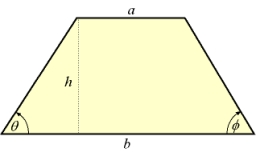# What is

What is the circumference of an isosceles trapezoid with a content of 106.75 cm 2 , the lengths of the sides are in the ratio 1: 3: 2: 1 and the bases are 6.1 cm apart?

cmDid you find an error or inaccuracy? Feel free to write us. Thank you!Tips to related online calculators
Check out our ratio calculator.
Do you have a linear equation or system of equations and looking for its solution? Or do you have a quadratic equation?

#### You need to know the following knowledge to solve this word math problem:

We encourage you to watch this tutorial video on this math problem: